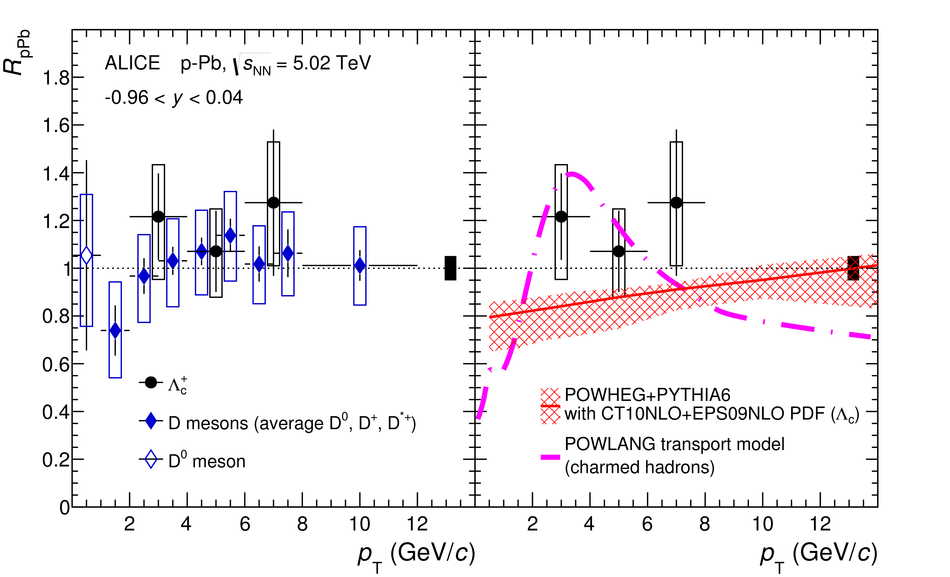# $Λ_{\rm c}^+$ production in pp collisions at $\sqrt{s} = 7$ TeV and in p-Pb collisions at $\sqrt{s_{\rm NN}} = 5.02$ TeV

The $p_{\rm T}$-differential production cross section of prompt $\Lambda_{\rm c}^+$ charmed baryons was measured with the ALICE detector at the Large Hadron Collider (LHC) in pp collisions at $\sqrt{s} = 7$ TeV and in p-Pb collisions at $\sqrt{s_{\rm NN}} = 5.02$ TeV at midrapidity. The $\Lambda_{\rm c}^+$ and ${\overline{\Lambda}}_{\rm c}^-$ were reconstructed in the hadronic decay modes $\Lambda_{\rm c}^+\rightarrow {\rm p}{\rm K^-}\pi^+$, $\Lambda_{\rm c}^+\rightarrow {\rm p}{\rm K_{\rm S}^0}$ and in the semileptonic channel $\Lambda_{\rm c}^+\rightarrow {\rm e^+}\nu_{\rm e}\Lambda$ (and charge conjugates). The measured values of the $\Lambda_{\rm c}^+/{\rm D_0}$ ratio, which is sensitive to the c-quark hadronisation mechanism, and in particular to the production of baryons, are presented and are larger than those measured previously in different colliding systems, centre-of-mass energies, rapidity and $p_{\rm T}$ intervals, where the $\Lambda_{\rm c}^+$ production process may differ. The results are compared with the expectations obtained from perturbative Quantum Chromodynamics calculations and Monte Carlo event generators. Neither perturbative QCD calculations nor Monte Carlo models reproduce the data, indicating that the fragmentation of heavy-flavour baryons is not well understood. The first measurement at the LHC of the $\Lambda_{\rm c}^+$ nuclear modification factor, $R_{\rm pPb}$, is also presented. The $R_{\rm pPb}$ is found to be consistent with unity and with that of D mesons within the uncertainties, and consistent with a theoretical calculation that includes cold nuclear matter effects and a calculation that includes charm quark interactions with a deconfined medium.

Figures

## Figure 1

 Proton identification with TPC (left) and TOF (right) in \pPb collisions. The discriminating PID variable $n_\sigma$ (see text for details) is shown as a function of the momentum $p$ of the particle. The $n_\sigma$ variable is computed assuming the proton hypothesis. The contributions from electrons and pions in the TPC and from kaons in the TOF are indicated.## Figure 2

 Normalised distribution of the BDT responses of the \Lc candidates for Monte Carlo signal (blue area) and background (red shaded area) in two \pt intervals for the \LctopKpi decay channel in \pPb collisions where the MVA method was used. The arrows correspond to the applied cuts.## Figure 3

 Invariant-mass distribution of $\Lcplus$ candidates (and charge conjugates) for $3 <$ \pt $< 4$ \gevc in pp collisions at \sqrts = 7~\tev. The dashed lines represent the fit to the background while the solid lines represent the total fit function. Left: $\LctopKpi$ STD analysis, right: $\LctopKzS$ STD analysis.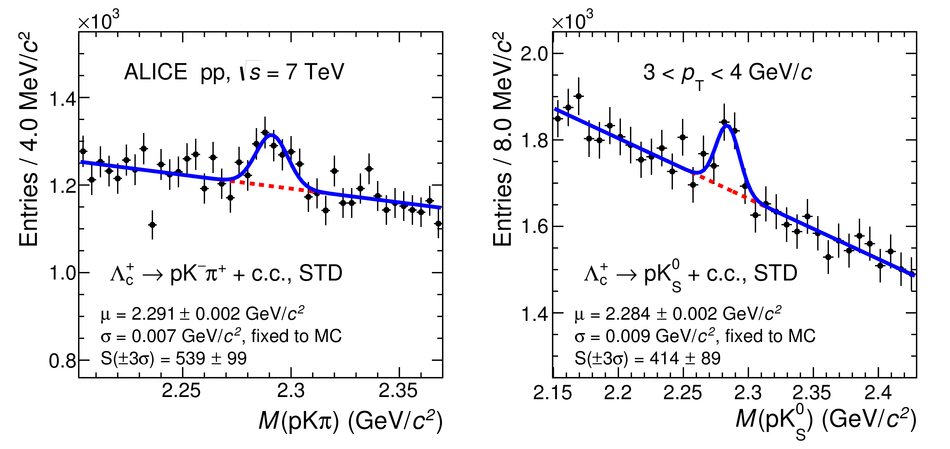## Figure 4

 Invariant-mass distribution of $\Lcplus$ candidates (and charge conjugates) for $4 <$ \pt $< 6$ \gevc in \pPb collisions at \sqrtsNN = 5.02~\tev. The dashed lines represent the fit to the background while the solid lines represent the total fit function. Top-left: $\LctopKpi$ STD analysis, top-right: $\LctopKpi$ MVA, bottom-left: $\LctopKzS$ STD analysis and bottom-right: $\LctopKzS$ MVA.## Figure 5

 Invariant mass distributions of e$\Lambda$ pairs for RS and WS combinations in the interval $3 < \pt^{{\rm e}\Lambda} < 4$ \gevc in pp collisions at $\sqrt{s}$~= 7 TeV.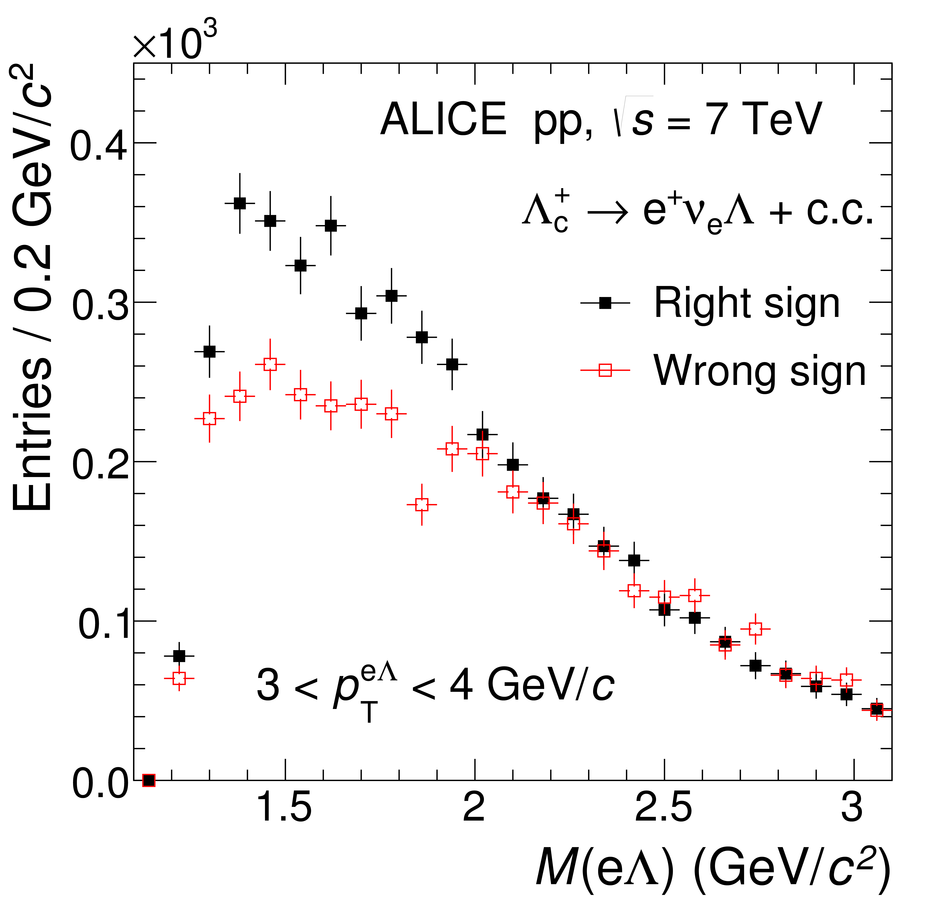## Figure 6

 Product of acceptance and efficiencyfor \Lc in pp collisions at $\sqrt{s}=7~\TeV$, as a function of \pt. From left to right: \LctopKpi, \LctopKzS, and \LctoenuLambda. For hadronic decays the solid lines correspond to the prompt \Lc, while the dotted lines represent $(A \times \epsilon)$ for \Lc baryons originating from beauty-hadron decays. The efficiency for semi-leptonic decays (same for both prompt and non-prompt \Lc) is represented with one solid line. The statistical uncertainties are smaller than the marker size.## Figure 7

 Product of acceptance and efficiency for the two \Lc hadronic decay channels in p-Pb collisions at \sqrtsNN = 5.02 \tev, as a function of \pt with the MVA technique. From left to right: \LctopKpi and \LctopKzS. The solid lines correspond to the prompt \Lc, while the dotted lines represent $(A \times \epsilon)$ for the \Lc from beauty-hadron decays. The statistical uncertainties are smaller than the marker size.## Figure 8

 Prompt \Lcplus~baryon $\pt$-differential production cross section in \pp collisions at \sqrts $= 7$~\tev in the transverse momentum interval $1 < \pt < 8$~\gevc (left) and in \pPb collisions at \sqrtsNN $= 5.02$ \tev in the transverse momentum interval $2 < \pt < 12$~\gevc (right). The statistical uncertainties are shown as error bars and the systematic uncertainties are shown as boxes. The markers for different analyses are shifted with respect tothe centre of the bin to improve visibility.## Figure 9

 Prompt \Lcplus~baryon $\pt$-differential cross section (average among different decay modes and analyses) in \pp collisions at \sqrts $= 7$ \tev in the transverse momentum interval $1 < \pt < 8$~\gevc (left) and in \pPb collisions at \sqrtsNN $= 5.02$ \tev in the transverse momentum interval $2 < \pt < 12$~\gevc (right). The statistical uncertainties are shown as error bars and the systematic uncertainties are shown as boxes. See text for details of the procedure to average the different decay channel measurements reported in Fig.~\ref{fig:CrossSection}. Comparisons with GM-VFNS calculations~, {\sc powheg} event generator~ and with Lansberg and Shao predictions~ for \pPb (see text for details) are also shown.## Figure 10

 The \Lcplus/\DZero ratio measured in \pp and \pPb collisions by ALICE, compared with the LHCb measurement~ as a function of \pt (left) and as a function of $y$ for $2 < \pt < 8$ \gevc (right).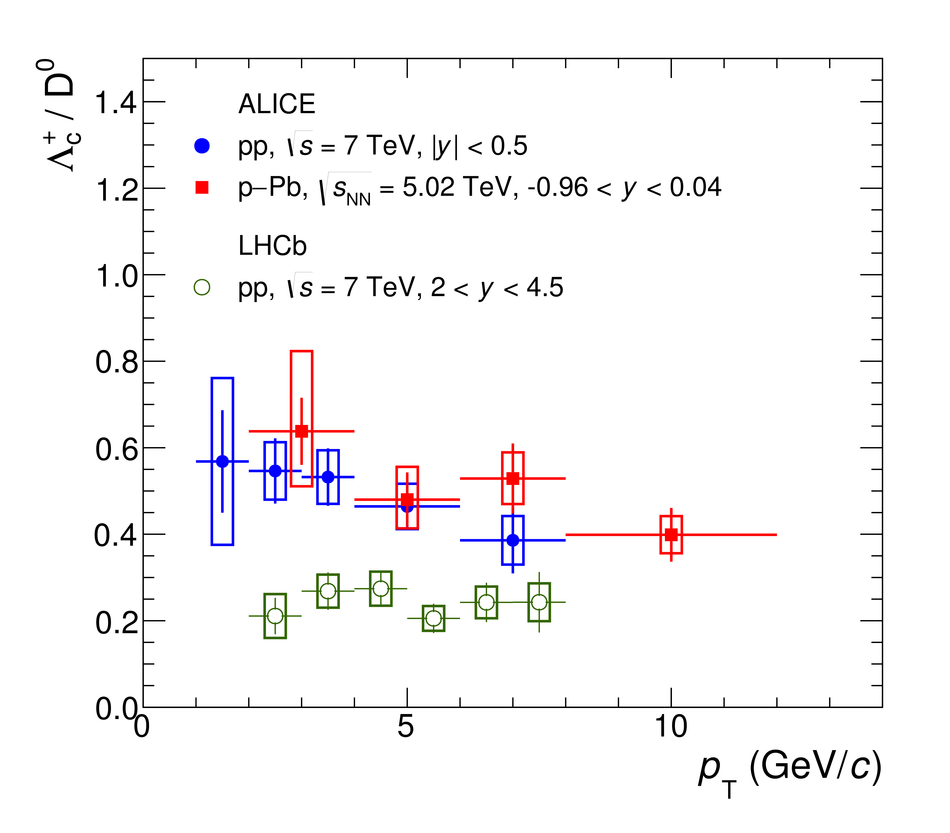## Figure 11

 The \Lcplus/\DZero ratio measured in \pp and \pPb collisions by ALICE as a function of \pt (left) and as a function of $y$ for $2 < \pt < 8$ \gevc (right). The measurements from \pp collisions are compared with different event generators (quoted tunes for {\sc pythia} and {\sc dipsy} taken respectively from~ and~). The \pPb measurement as a function of \pT is also compared with calculations from Lansberg and Shao~. The predictions from event generators as a function of $y$ are also compared with the LHCb measurement~.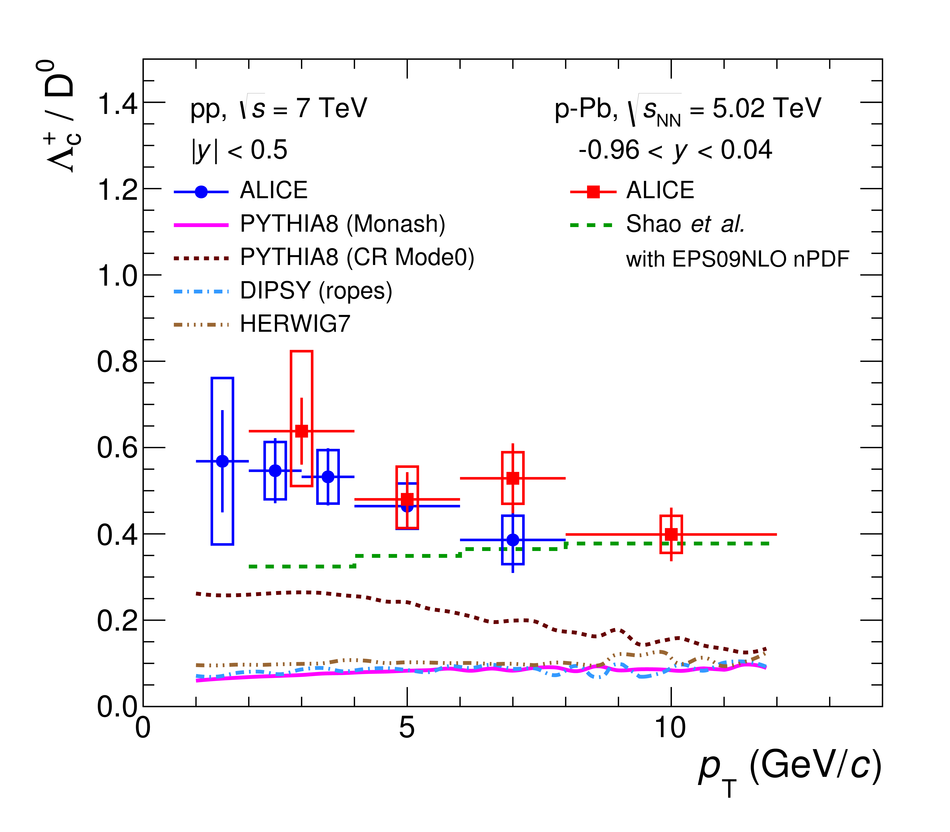## Figure 12

 The nuclear modification factor \RpPb of prompt \Lcplus~baryons in \pPb collisions at \sqrtsNN = 5.02 TeV as a function of \pT compared to that of D mesons (average of \Dzero, \Dplus and \Dstar in the range $1 < \pt < 12$~\gevc, and \Dzero in the range $0 < \pt < 1$~\gevc)~ (left panel) and to model calculations (right panel). The predictions for the comparison are the \Lcplus~\RpPb~from the {\sc powheg} event generator~ with EPS09NLO parameterisation of the nuclear modification of the PDFs~ and the charm-hadron \RpPb from the POWLANG transport model~ assuming a QGP is formed in \pPb collisions.